Quandaries and Queries We can't figure this out. 10th grade geometry. Student and stupid parent. This shape would occur at the four corners of a rectangular shaped earthen pit with sloping sides (1:1.5). Depth is 14'. Top dimensions are 170' by 158'. After calculating the easy volume components of this shape, we are left with the end corner pieces. 21' base, 14' height, side hypotenuse 25.24' and corner diagonal 32.83'. We're confused. Thank you for any help you may provide. Regards Hi, I would approach this problem in a different way. Imagine that the earthen pit is a solid block of something like butter, sitting on a table.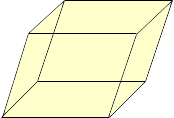Slice the end off by a cut which is perpendicular to the table.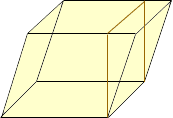Move the piece sliced off to the other end and, since the slopes of the two ends were the same, it will fit nicely into place.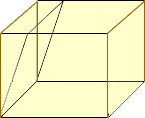Now rotate the block 90o to look at the end. (you have to use some imagination here as my drawing ability is minimal.)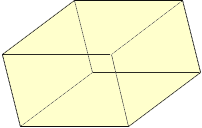Again slice off the end by a cut which is perpendicular to the table,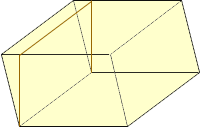and slide the piece cut off to the other end.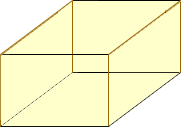This process does not change the volume of the block, it only changes its configuration. What you have now is a rectangular block with its base the same dimensions as the original block and its height the same height as the original block. Its volume is the length times the width times the height so the volume of the earthen pit is 17015814 cubic feet. Penny Go to Math Central